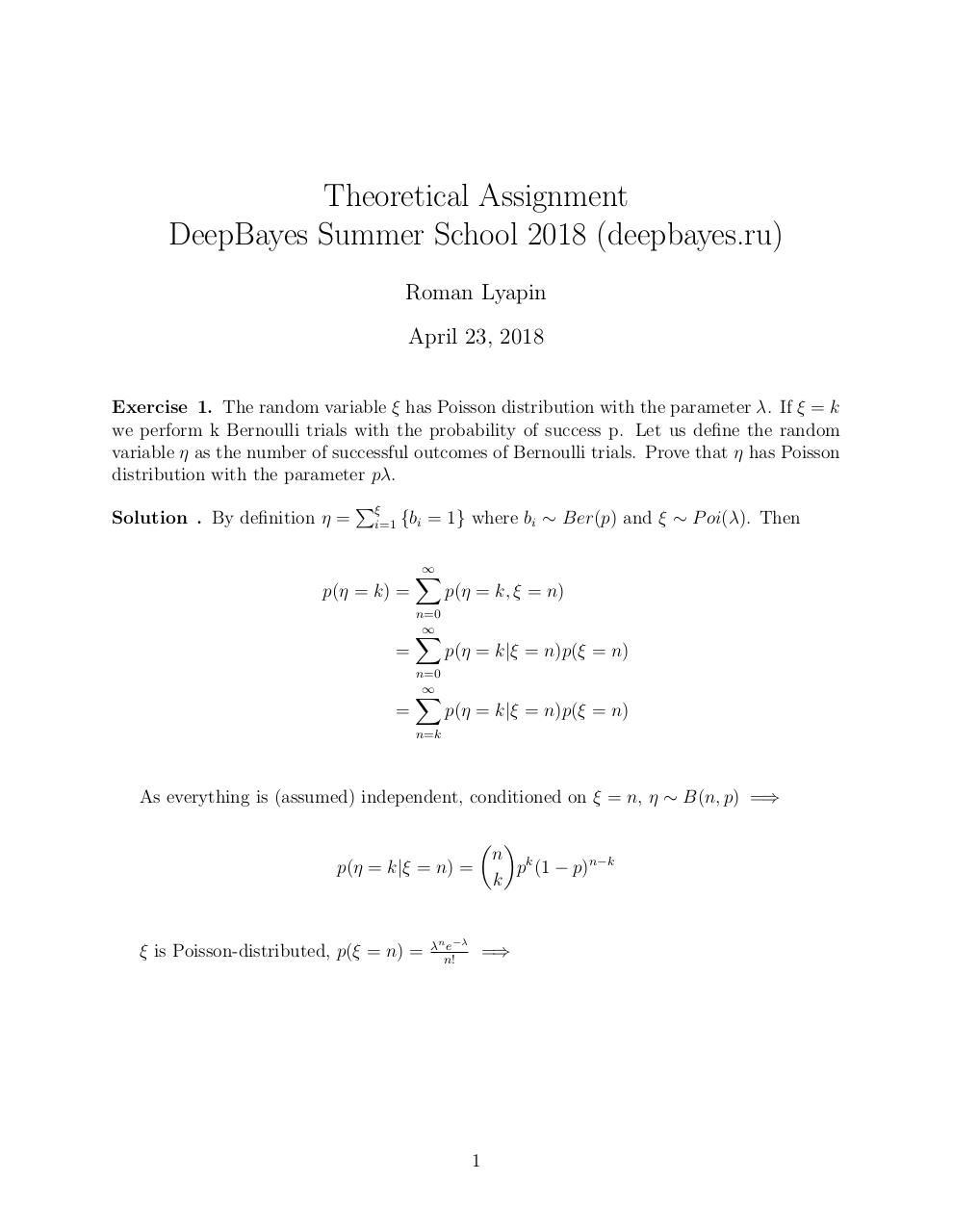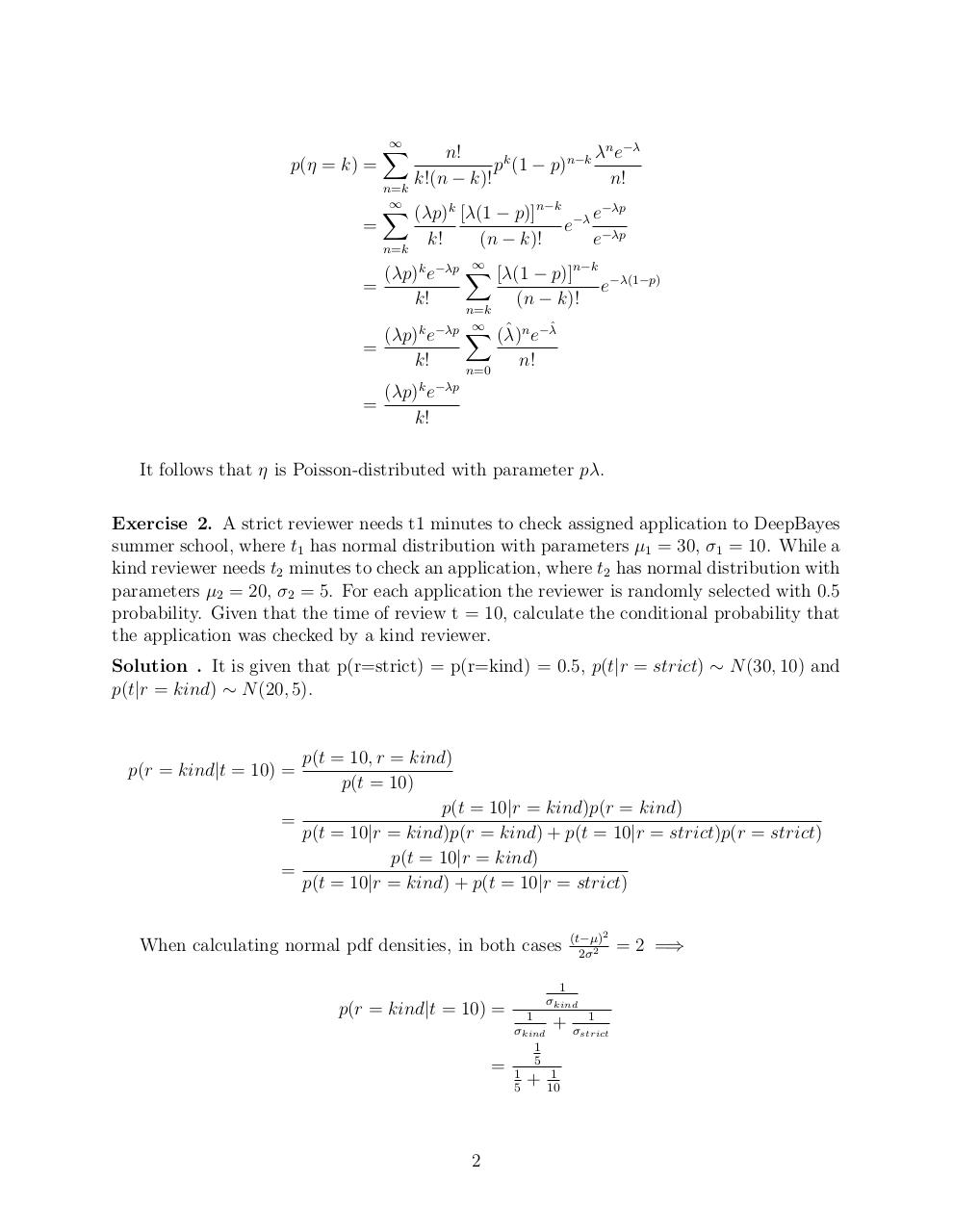# RomanLyapin TheoryAssignment .pdf

### File information

Original filename: RomanLyapin_TheoryAssignment.pdf

This PDF 1.5 document has been generated by TeX / pdfTeX-1.40.17, and has been sent on pdf-archive.com on 24/04/2018 at 16:34, from IP address 5.57.x.x. The current document download page has been viewed 538 times.
File size: 119 KB (3 pages).
Privacy: public file

RomanLyapin_TheoryAssignment.pdf (PDF, 119 KB)

### Document preview

Theoretical Assignment
DeepBayes Summer School 2018 (deepbayes.ru)
Roman Lyapin
April 23, 2018
Exercise 1. The random variable ξ has Poisson distribution with the parameter λ. If ξ = k
we perform k Bernoulli trials with the probability of success p. Let us define the random
variable η as the number of successful outcomes of Bernoulli trials. Prove that η has Poisson
distribution with the parameter pλ.
Solution . By definition η =

i=1

{bi = 1} where bi ∼ Ber(p) and ξ ∼ P oi(λ). Then

p(η = k) =

X

p(η = k, ξ = n)

n=0

=
=

X
n=0

X

p(η = k|ξ = n)p(ξ = n)
p(η = k|ξ = n)p(ξ = n)

n=k

As everything is (assumed) independent, conditioned on ξ = n, η ∼ B(n, p) =⇒
 
n k
p(η = k|ξ = n) =
p (1 − p)n−k
k

ξ is Poisson-distributed, p(ξ = n) =

λn e−λ
n!

=⇒

1

p(η = k) =
=

X
n=k

X
n=k

=

λn e−λ
n!
pk (1 − p)n−k
k!(n − k)!
n!
(λp)k [λ(1 − p)]n−k −λ e−λp
e
k!
(n − k)!
e−λp

(λp)k e−λp X [λ(1 − p)]n−k −λ(1−p)
e
k!
(n

k)!
n=k

ˆ n e−λˆ
(λp)k e−λp X (λ)
=
k!
n!
n=0

=

(λp)k e−λp
k!

It follows that η is Poisson-distributed with parameter pλ.
Exercise 2. A strict reviewer needs t1 minutes to check assigned application to DeepBayes
summer school, where t1 has normal distribution with parameters µ1 = 30, σ1 = 10. While a
kind reviewer needs t2 minutes to check an application, where t2 has normal distribution with
parameters µ2 = 20, σ2 = 5. For each application the reviewer is randomly selected with 0.5
probability. Given that the time of review t = 10, calculate the conditional probability that
the application was checked by a kind reviewer.
Solution . It is given that p(r=strict) = p(r=kind) = 0.5, p(t|r = strict) ∼ N (30, 10) and
p(t|r = kind) ∼ N (20, 5).

p(t = 10, r = kind)
p(t = 10)
p(t = 10|r = kind)p(r = kind)
=
p(t = 10|r = kind)p(r = kind) + p(t = 10|r = strict)p(r = strict)
p(t = 10|r = kind)
=
p(t = 10|r = kind) + p(t = 10|r = strict)

p(r = kind|t = 10) =

When calculating normal pdf densities, in both cases

p(r = kind|t = 10) =
=

2

(t−µ)2
2σ 2

1
σkind
1
1
+ σstrict
σkind
1
5
1
1
+
5
10

= 2 =⇒

It follows that the probability the application was reviewed by kind reviewer is 23 .

3Use the permanent link to the download page to share your document on Facebook, Twitter, LinkedIn, or directly with a contact by e-Mail, Messenger, Whatsapp, Line..

Use the short link to share your document on Twitter or by text message (SMS)

#### HTML Code

Copy the following HTML code to share your document on a Website or Blog

#### QR Code### Related keywords